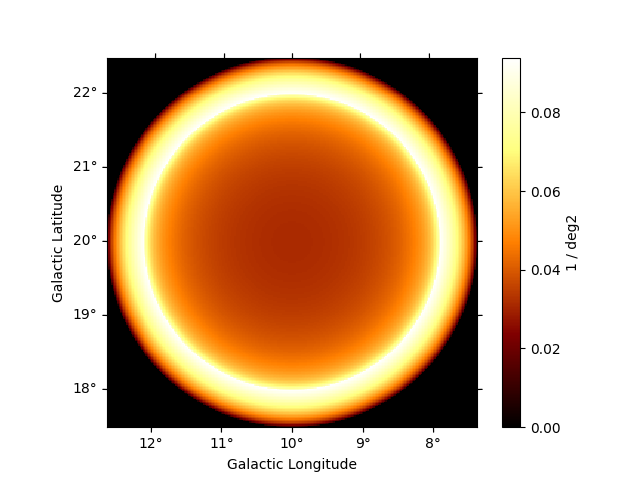# Shell spatial model#

This is a spatial model parametrizing a projected radiating shell.

The shell spatial model is defined by the following equations:

$\begin{split}\phi(lon, lat) = \frac{3}{2 \pi (r_{out}^3 - r_{in}^3)} \cdot \begin{cases} \sqrt{r_{out}^2 - \theta^2} - \sqrt{r_{in}^2 - \theta^2} & \text{for } \theta \lt r_{in} \\ \sqrt{r_{out}^2 - \theta^2} & \text{for } r_{in} \leq \theta \lt r_{out} \\ 0 & \text{for } \theta > r_{out} \end{cases}\end{split}$

where $$\theta$$ is the sky separation and $$r_{\text{out}} = r_{\text{in}}$$ + width

Note that the normalization is a small angle approximation, although that approximation is still very good even for 10 deg radius shells.

## Example plot#

Here is an example plot of the model:

from gammapy.modeling.models import (
Models,
PowerLawSpectralModel,
ShellSpatialModel,
SkyModel,
)

model = ShellSpatialModel(
lon_0="10 deg",
lat_0="20 deg",
width="0.5 deg",
frame="galactic",
)<WCSAxes: >


## YAML representation#

Here is an example YAML file using the model:

pwl = PowerLawSpectralModel()
shell = ShellSpatialModel()

model = SkyModel(spectral_model=pwl, spatial_model=shell, name="pwl-shell-model")
models = Models([model])

print(models.to_yaml())

components:
-   name: pwl-shell-model
type: SkyModel
spectral:
type: PowerLawSpectralModel
parameters:
-   name: index
value: 2.0
-   name: amplitude
value: 1.0e-12
unit: cm-2 s-1 TeV-1
-   name: reference
value: 1.0
unit: TeV
spatial:
type: ShellSpatialModel
frame: icrs
parameters:
-   name: lon_0
value: 0.0
unit: deg
-   name: lat_0
value: 0.0
unit: deg